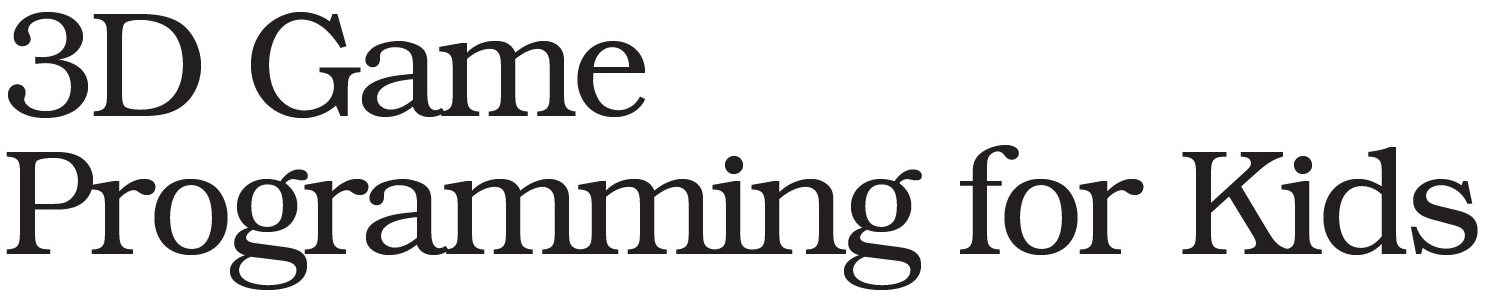# Have a question and a request.

I’m still using the 2013 version, which is the first edition of the book. Unfortunately, the source code on the pragmatic bookshelf website is for the second edition, which does not work in ICE for some reason, even if I directly copy/paste it. Opening the console shows a lot of “Failed to load resource: the server responded with a status of 404 (Not Found)” errors, and a few “Uncaught ReferenceError: THREE is not defined” errors. Help! Here is the directly copy-pasted code.
By the way, my request is the original source code for the first edition. This is the moon phases file.

``````
<body></body>
<script src="/three.js"></script>
<script src="/controls/FlyControls.js"></script>
<script>
// The "scene" is where stuff in our game will happen:
var scene = new THREE.Scene();
var light = new THREE.AmbientLight('white', 0.1);

// The "camera" is what sees the stuff:
var aspectRatio = window.innerWidth / window.innerHeight;
var w = window.innerWidth / 2;
var h = window.innerHeight / 2;
var camera = new THREE.OrthographicCamera(-w, w, h, -h, 1, 10000);
camera.position.y = 500;
camera.rotation.x = -Math.PI/2;
var aboveCam = camera;

// The "renderer" draws what the camera sees onto the screen:
var renderer = new THREE.WebGLRenderer({antialias: true});
renderer.setSize(window.innerWidth, window.innerHeight);
document.body.appendChild(renderer.domElement);

// ******** START CODING ON THE NEXT LINE ********

var cover = new THREE.MeshPhongMaterial({emissive: 'yellow'});
var shape = new THREE.SphereGeometry(50, 32, 16);
var sun = new THREE.Mesh(shape, cover);

var sunlight = new THREE.PointLight('white', 1.7);

var earthLocal = new THREE.Object3D();
earthLocal.position.x = 300;

var cover = new THREE.MeshPhongMaterial({map: texture});
var shape = new THREE.SphereGeometry(20, 32, 16);
var earth = new THREE.Mesh(shape, cover);

var moonOrbit = new THREE.Object3D();

var cover = new THREE.MeshPhongMaterial({map: texture, specular: 'black'});
var shape = new THREE.SphereGeometry(15, 32, 16);
var moon = new THREE.Mesh(shape, cover);
moon.position.set(0, 0, 100);
moon.rotation.set(0, Math.PI/2, 0);

var moonCam = new THREE.PerspectiveCamera(70, aspectRatio, 1, 10000);
moonCam.position.z = 25;
moonCam.rotation.y = Math.PI;

camera = moonCam;

var shipCam = new THREE.PerspectiveCamera(75, aspectRatio, 1, 10000);
shipCam.position.set(0, 0, 500);

var controls = new THREE.FlyControls(shipCam, renderer.domElement);
controls.movementSpeed = 42;
controls.rollSpeed = 0.15;
controls.dragToLook = true;
controls.autoForward = false;

var cover = new THREE.PointsMaterial({color: 'white', size: 15});
var shape = new THREE.Geometry();

var distance = 4000;
for (var i = 0; i < 500; i++) {
var ra = 2 * Math.PI * Math.random();
var dec = 2 * Math.PI * Math.random();

var point = new THREE.Vector3();
point.x = distance * Math.cos(dec) * Math.cos(ra);
point.y = distance * Math.sin(dec);
point.z = distance * Math.cos(dec) * Math.sin(ra);

shape.vertices.push(point);
}

var stars = new THREE.Points(shape, cover);

// Start Animation

var clock = new THREE.Clock();
function animate() {
requestAnimationFrame(animate);

// Animation code goes here...
var delta = clock.getDelta();
controls.update(delta);

renderer.render(scene, camera);
}
animate();

var speed = 10;
var pause = false;
var days = 0;
var clock2 = new THREE.Clock();
function gameStep() {
setTimeout(gameStep, 1000/30);

if (pause) return;

days = days + speed * clock2.getDelta();

earth.rotation.y = days;

var years = days / 365.25;
earthLocal.position.x = 300 * Math.cos(years);
earthLocal.position.z = -300 * Math.sin(years);
moonOrbit.rotation.y = days / 29.5;
}
gameStep();

function sendKeyDown(event) {
var code = event.code;

if (code == 'Digit1') speed = 1;
if (code == 'Digit2') speed = 10;
if (code == 'Digit3') speed = 100;
if (code == 'Digit4') speed = 1000;
if (code == 'KeyP') pauseUnpause();
if (code == 'KeyC') switchCamera();
if (code == 'KeyF') fly();
}

function pauseUnpause() {
pause = !pause;
clock2.running = false;
}

function switchCamera() {
if (camera == moonCam) camera = aboveCam;
else camera = moonCam;
}

function fly() {
camera = shipCam;
}
</script>
``````

Sorry for the trouble! The source code for the first edition of the book is located at: https://media.pragprog.com/titles/csjava/code/csjava-code.zip.

The source for the second edition will definitely not work with the first. Many of the libraries have been replaced.

-Chris